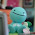## Friday, January 14, 2011

### Final Percent Post

Percent
a percent means out of 100
a percent can be represented as a fraction, decimal and in a hundred grid

Key Points of Chapter 4
4.1

When representing a percent greater than 100%, use more than 1 grid.
When representing a percent less than 1%, but more than zero, shade a part of one square.
4.2
Percents can be represented as a fraction, and a decimal.
1/2 = 50% = 0.50
4.3
To calculate a percent of a number, write the percent as a decimal then multiply the number
12 1/2% of 50= 0.125x50
= 6.25
4.4
To combine a percent, add them together.
To found out the increase in a number, add the combined percent to the original number.
5% + 7%(taxes) 12%

My percent video:

My Percent Scribe

1.